• +91 9971497814
• info@interviewmaterial.com

# RD Chapter 6- Graphs of Trigonometric Functions Ex-6.2 Interview Questions Answers

### Related Subjects

Question 1 :
Sketch the graphs of the following trigonometric functions:
(i) f (x) = cos (x – π/4)
(ii) g (x) = cos (x + π/4)
(iii) h (x) = cos2 2x
(iv) ϕ (x) = 2 cos (x – π/6)
(v) ψ (x) = cos (3x)
(vi) u (x) = cos2 x/2
(vii) f (x) = cos πx
(viii) g (x) = cos 2π x

(i) f (x) = cos (x – π/4)
We know that g (x) = cos x is a periodic function with period 2π.
So, f (x) = cos (x – π/4) is a periodic function with period π. So, we will draw the graph of f (x) = cos (x – π/4) in the interval [0, π]. The values of f (x) = cos (x – π/4) at various points in [0, π] are listed in the following table:
 x 0 (A) π/4 (B) π/2 (C) 3π/4 (D) π (E) 5π/4 (F) 3π/2 (G) 7π/4 (H) f (x) = cos (x – π/4) 1/√2 = 0.7 1 1/√2 = 0.7 0 -1/√2 = -0.7 -1 -1/√2 = -0.7 0

The required curve is: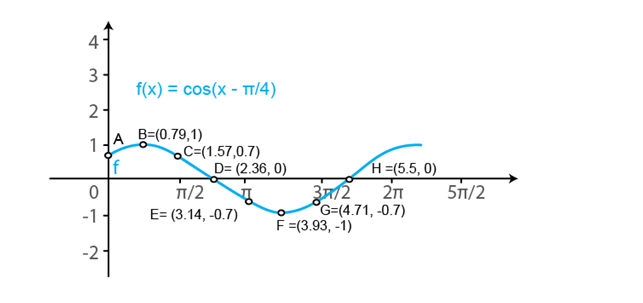(ii) g (x) = cos (x + π/4)

We know that f (x) = cos x is a periodic function with period 2π.

So, g (x) = cos (x + π/4) is a periodic function with period π. So, we will draw the graph of g (x) = cos (x + π/4) in the interval [0, π]. The values of g (x) = cos (x + π/4) at various points in [0, π] are listed in the following table:

 x 0 (A) π/4 (B) π/2 (C) 3π/4 (D) π (E) 5π/4 (F) 3π/2 (G) 7π/4 (H) g (x) = cos (x + π/4) 1/√2 = 0.7 0 -1/√2 = -0.7 -1 -1/√2 = -0.7 0 1/√2 = 0.7 1

The required curve is: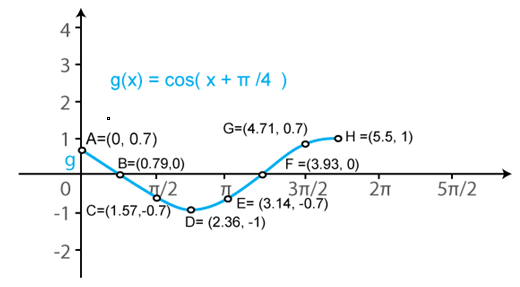(iii) h (x) = cos2 2x

We know that f (x) = cos x is a periodic function withperiod 2π.

So, h (x) = cos2 2x is a periodicfunction with period π. So, we will draw the graph of h (x) = cos2 2xin the interval [0, π]. The values of h (x) = cos2 2x atvarious points in [0, π] are listed in the following table:

 x 0 (A) π/4 (B) π/2 (C) 3π/4 (D) π (E) 5π/4 (F) 3π/2 (G) h (x) = cos2 2x 1 0 1 0 1 0 1

The required curve is: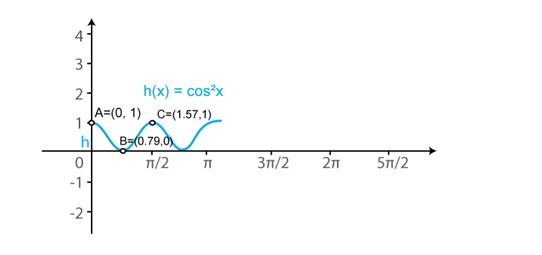(iv) ϕ (x) = 2 cos (x – π/6)

We know that f (x) = cos x is a periodic function with period 2π.

So, ϕ (x) = 2cos (x – π/6) is a periodic function with period π. So, we will draw the graph of ϕ (x) = 2cos (x – π/6) in the interval [0, π]. The values of ϕ (x) = 2cos (x – π/6) at various points in [0, π] are listed in the following table:

 x 0 (A) π/3 (B) 2π/3 (C) π (D) 4π/3 (E) 5π/3 (F) ϕ (x) = 2 cos (x – π/6) √3 = 1.73 √3 = 1.73 0 -√3 = -1.73 -√3 = -1.73 0

The required curve is: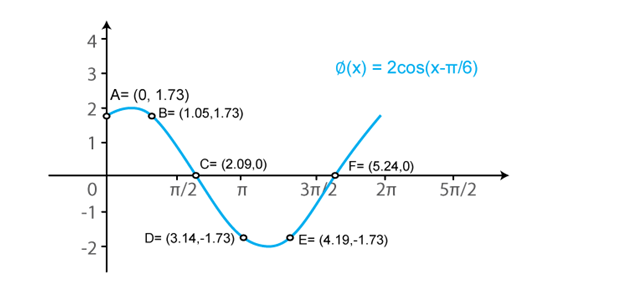(v) ψ (x) = cos (3x)

We know that f (x) = cos x is a periodic function with period 2π.

So, ψ (x) = cos (3x) is a periodic function with period 2π/3. So, we will draw the graph of ψ (x) = cos (3x) in the interval [0, 2π/3]. The values of ψ (x) = cos (3x) at various points in [0, 2π/3] are listed in the following table:

 x 0 (A) π/6 (B) π/3 (C) π/2 (D) 2π/3 (E) 5π/6 (F) ψ (x) = cos (3x) 1 0 -1 0 1 0

The required curve is: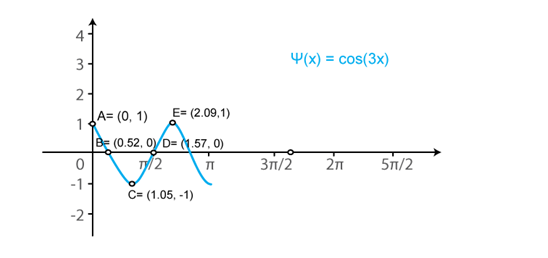(vi) u (x) = cos2 x/2

We know that f (x) = cos x is a periodic function withperiod 2π.

So, u (x) = cos2 (x/2) is a periodicfunction with period π. So, we will draw the graph of u (x) = cos2 (x/2)in the interval [0, π]. The values of u (x) = cos2 (x/2) atvarious points in [0, π] are listed in the following table:

 x 0 (A) π (B) 2π (C) 3π (D) u (x) = cos2 x/2 1 0 1 0

The required curve is: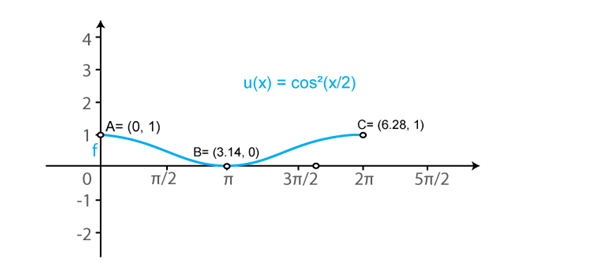(vii) f (x) = cos πx

We know that g (x) = cos x is a periodic function withperiod 2π.

So, f (x) = cos (πx) is a periodic function withperiod 2. So, we will draw the graph of f (x) = cos (πx) in the interval [0,2]. The values of f (x) = cos (πx) at various points in [0, 2] are listed inthe following table:

 x 0 (A) 1/2 (B) 1 (C) 3/2 (D) 2 (E) 5/2 (F) f (x) = cos πx 1 0 -1 0 1 0

The required curve is: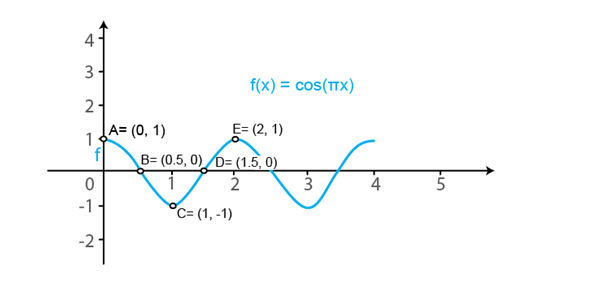(viii) g (x) = cos 2π x

We know that f (x) = cos x is a periodic function withperiod 2π.

So, g (x) = cos (2πx) is a periodic function withperiod 1. So, we will draw the graph of g (x) = cos (2πx) in the interval [0,1]. The values of g (x) = cos (2πx) at various points in [0, 1] are listed inthe following table:

 x 0 (A) 1/4 (B) 1/2 (C) 3/4 (D) 1 (E) 5/4 (F) 3/2 (G) 7/4 (H) 2 g (x) = cos 2π x 1 0 -1 0 1 0 -1 0 1

The required curve is: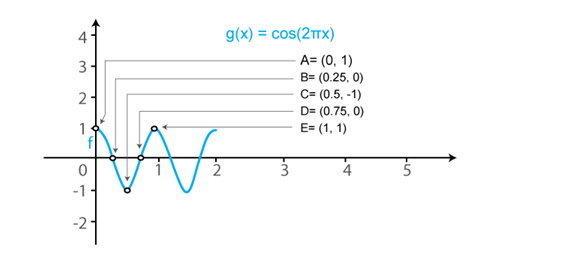Question 2 :
Sketch the graphs of the following curves on the same scale and the same axes:
(i) y = cos x and y = cos (x – π/4)
(ii) y = cos 2x and y = cos (x – π/4)
(iii) y = cos x and y = cos x/2
(iv) y = cos2 x and y = cos x

(i) y = cos x and y = cos (x – π/4)
We know that the functions y = cos x and y = cos (x – π/4) are periodic functions with periods π and π.
The values of these functions are tabulated below:
 x 0 π/4 π/2 3π/4 π 5π/4 3π/2 7π/4 y = cos x 1 1/√2 = 0.7 0 -1/√2 = -0.7 -1 -1/√2 = -0.7 0 1 y = cos (x – π/4) 1/√2 = 0.7 1 1/√2 = 0.7 0 -1/√2 = -0.7 -1 -1/√2 = -0.7 0

The required curve is: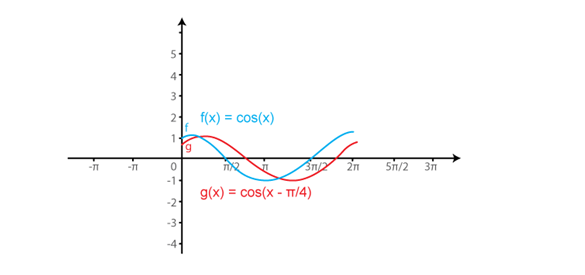(ii) y = cos 2x and y = cos 2(x – π/4)

We know that the functions y = cos 2x and y = cos 2(x – π/4) are periodic functions with periods π and π.

The values of these functions are tabulated below:

 x 0 π/4 π/2 3π/4 π 5π/4 3π/2 7π/4 y = cos x 1 0 -1 0 1 0 -1 0 y = cos 2 (x – π/4) 0 1 0 -1 0 1 0 -1

The required curve is: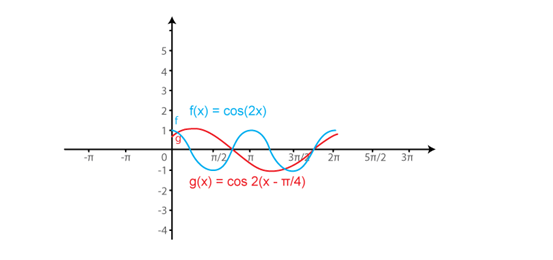(iii) y = cos x and y = cos x/2

We know that the functions y = cos x and y = cos (x/2) are periodic functions with periods π and π.

The values of these functions are tabulated below:

 x 0 π/2 π 3π/2 2π y = cos x 1 0 -1 0 1 y = cos x/2 1 1/√2 = 0.7 0 -1/√2 = -0.7 -1

The required curve is: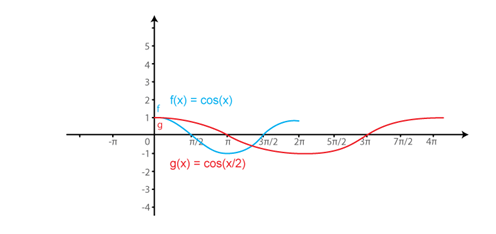(iv) y = cos2 x and y = cos x

We know that the functions y = cos2 xand y = cos x are periodic functions with period 2π.

The values of these functions are tabulated below:

 x 0 π/2 π 3π/2 2π y = cos2 x 1 0 1 0 1 y = cos x 1 0 -1 0 1

The required curve is: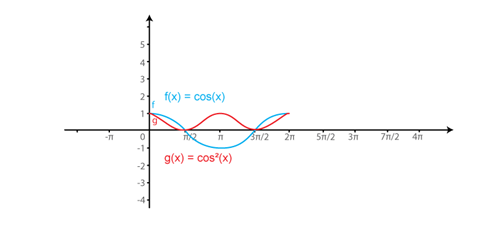Todays Deals### RD Chapter 6- Graphs of Trigonometric Functions Ex-6.2 Contributorskrishan

Name:
Email:

# Latest News# 9000 interview questions in different categories